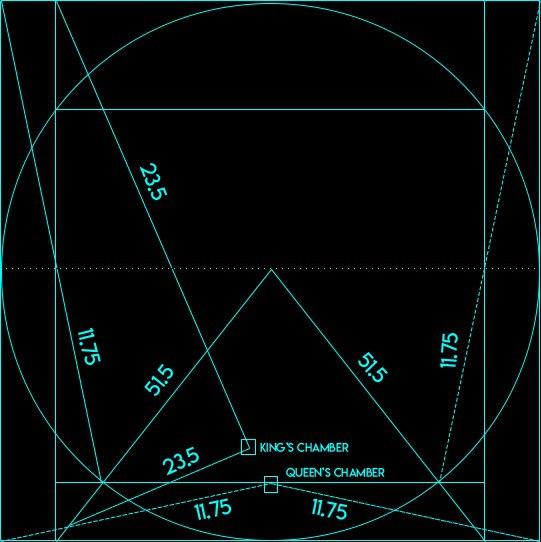# Numbers To Study

The numbers that need your attention are math constants.

The more you see them and connect with them the more they will begin to appear.

Below is a list of these math constants.

Look at these numbers constantly then look for them everywhere.

Tweets, emails, receipts, timestamps, prices, dates, on your charts… Heck, even the numbers on the dashboard of your car.

Look at the numbers given to you and when they are given to you.

What is going on around you?

What message is being sent to you?

## Great Pyramid of Giza

Below is the chambers.

Notice the angles.

## Piπ

This mathematical constant known as pi has a value of 3.14 and has a strong relationship with geometry.

Specifically circles, spheres, and ellipses.

Usually denotes a cycle change happening… Something beginning anew, or completing.

Examine the image below.

Using Vortex Math, we see that all numbers hold a vibration even when rearranged in the order represented.

For example, PI being 3.14… The variants are anything with those numbers.

413, 431, 341, 134, 143 all carry the same energy/vibration of 8

## Phi Φ

This mathematical constant is also known as the golden ratio with a value of 1.618

It is found in nature and has been implemented in art, architecture, and charts!

Phi is typically associate with expansive growth or contraction.

Remember, using Vortex Math, all phi numbers hold a vibration to them even when rearranged in the order represented.

For example, PHI being 1.618 the variants are anything with those numbers 618, 168, 186, 681, 816, 861 all carry the same energy/vibration

## SOL

Check the article here on SOL and why 144 is the speed of light (SOL).

SOL is the guide rails for Phi. The places where Time and Speed interact.

Vortex Math applies here too.

All of the numbers listed as well as the variants as they carry the same vibrational value.

SOL being 144 the variants are anything with those numbers 141, 114, 411, 414, 441

## 369

This is the divine cycle inside of the Vortex Math.

The angles complete the triangle that forms, which is also tied to a cycle completion or can also be used as a confirmation on a higher level of something happening or coming to fruition.

Look at the Gann emblem again… Do you see it differently now?

A circle (pi) containing a square(when bisected is 2 triangles) and a triangle (369) also 3 – 120° angles. (120 = 3)

3 is the magic number